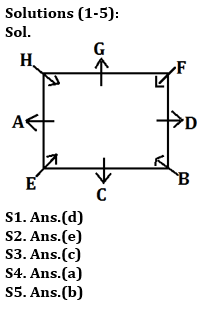Latest Banking jobs   »

# Reasoning Quiz For ECGC PO 2023 -12th May

Directions (1-5): Study the given information and answer the questions:
Eight friends A, B, C, D, E, F, G and H are sitting around a square table in such a way that four of them sit at the four corners while four sits at the middle of each of four sides. The one who sit at the corners face the center and who sit at the middle of the sides face outwards but not necessarily in the same order.
F sits third to left of C. C faces outward. H sits immediate right of A. F sits second to right of B. E sits on the immediate left of A. D is not immediate neighbor of H. Only two friends sit between F and A.

Q1. Who among the following sits at corner of the table?
(a) C
(b) D
(c) G
(d) B
(e) None of these

Q2. Who among the following sits opposite to D?
(a) G
(b) F
(c) C
(d) B
(e) A

Q3. Who among the following sits immediate left of B?
(a) A
(b) E
(c) C
(d) G
(e) None of these

Q4. Who among the following sits 2nd to the left of G?
(a) A
(b) H
(c) B
(d) E
(e) F

Q5. Who among following sits opposite to H?
(a) E
(b) B
(c) F
(d) A
(e) None of these

Directions (6-10): In number arrangement machine when given an input line of numbers, it rearranges them following a particular rule in each step. The following is an illustration of input and rearrangement.

Input: 12 75 28 36 93 44 51
Step I: 13 72 29 37 90 45 48
Step II: 90 72 48 45 37 29 13
Step III: 9 5 4 1 4 7 2
Step IV: 18 10 12 2 12 14 6

And step IV is the last step of the arrangement. As per the above rule followed in the above steps, find out in each of the following questions the appropriate step for the input given below;

Input: 86 17 57 26 65 43 72

Q6. Which of the following digit is fifth from the right end in step-III?
(a) 4
(b) 1
(c) 5
(d) 3
(e) None of these

Q7. What is the sum of the numbers which are second from the left end in step-II and third to the right end in step IV?
(a) 21
(b) 85
(c) 87
(d) 56
(e) None of these

Q8. How many prime numbers are obtained in last step?
(a) Four
(b) One
(c) Two
(d) Three
(e) None

Q9. What is the multiplication of the digits of the number which is third from the right end in step II?
(a) 18
(b) 10
(c) 21
(d) 25
(e) None of these

Q10. What is the difference between the numbers which is third from the left end in last step and second from the right end in first step?
(a) 7
(b) 27
(c) 31
(d) 40
(e) None of these

SolutionsSolutions (6-10):
Sol. Let us understand the logic behind it-
* In Step-1, one is added in each even digit and three is subtracted from each odd digit of the input.
* In Step-2, All the numbers of step-1 arranged in descending order from left to right.
* In Step-3, Digits of the number are subtracted with in the number which are obtained in step-2.
* In Step-4, two is multiplied in each odd digit and three is multiplied in each even digit which are obtained in step-3.

Input: 86 17 57 26 65 43 72
Step I: 87 14 54 27 62 40 73
Step II: 87 73 62 54 40 27 14
Step III: 1 4 4 1 4 5 3
Step IV: 2 12 12 2 12 10 6

S6. Ans.(a)
S7. Ans.(b)
S8. Ans.(c)
S9. Ans.(e)
S10. Ans.(e)## FAQs

### What is the total duration of ECGC PO for objective?

The duration of ECGC PO for objective is 140 minutes

#### Congratulations!Union Budget 2023-24: Free PDF